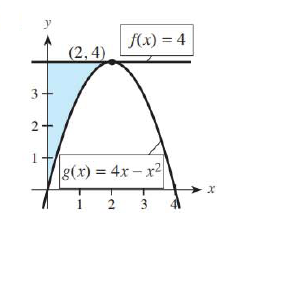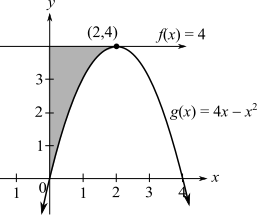Chapter 13.3, Problem 2E### Mathematical Applications for the ...

11th Edition
Ronald J. Harshbarger + 1 other
ISBN: 9781305108042

#### Solutions

Chapter
Section### Mathematical Applications for the ...

11th Edition
Ronald J. Harshbarger + 1 other
ISBN: 9781305108042
Textbook Problem

# For each shaded region in Problems 1-6, (a) form the integral that represents the area of the shaded region and (b) find the area of the region.(a)

To determine

To calculate: The integral that represents the area of the shaded region.Explanation

Given information:

The provided region is:

Formula used:

Area between two Curves:

If f and g are continuous functions on [a,b] and if f(x)g(x) for all x in [a,b] then the area of the region between the graphs of f and g are given and between x=a and x=b is given by:

A=ab[f(x)g(x)dx]

Calculation:

Consider the curve:

It can be deduced from the above graph that f(x)g(x) and the range is from x=0 to x=2

Consider the formula:

A=ab[f(x)

(b)

To determine

To calculate: The area of the region### Still sussing out bartleby?

Check out a sample textbook solution.

See a sample solution

#### The Solution to Your Study Problems

Bartleby provides explanations to thousands of textbook problems written by our experts, many with advanced degrees!

Get Started

#### Find more solutions based on key concepts